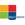# Activities

••• ##### Subject Area

• Math: Geometry: Points, Lines and Angles

• ##### Author6-8
9-12

45 Minutes

• ##### Device
• TI-83 Plus Family
• TI-84 Plus
• TI-84 Plus Silver Edition
• ##### Other Materials
For more information on the Holt, Rinehart and Winston Mathematics Series, visit them on the web at www.hrw.com/math

## Explore Parallel and Perpendicular Lines

#### Activity Overview

Students will use a graphing calculator to explore graphs of parallel and perpendicular lines. Students will find that parallel lines have the same slope and different y-intercepts and that the slopes of two perpendicular lines are opposite reciprocals of each other.

This Technology Lab accompanies Lesson 3-6 from the ©2007 Holt, Rinehart and Winston Geometry textbook.

#### Before the Activity

• Review the lesson PDF files.
• Make copies of the lesson PDF files for your class.
• #### During the Activity

• Students will use a graphing calculator to explore graphs of parallel and perpendicular lines.
• Students will learn the slope-intercept form of the equation of a line.
• Students will find that parallel lines have the same slope and different y-intercepts.
• Students will learn that the slopes of two perpendicular lines are opposite reciprocals of each other.
• #### After the Activity

Review student results:

• As a class, discuss questions that appeared to be more challenging
• Re-teach concepts as necessary